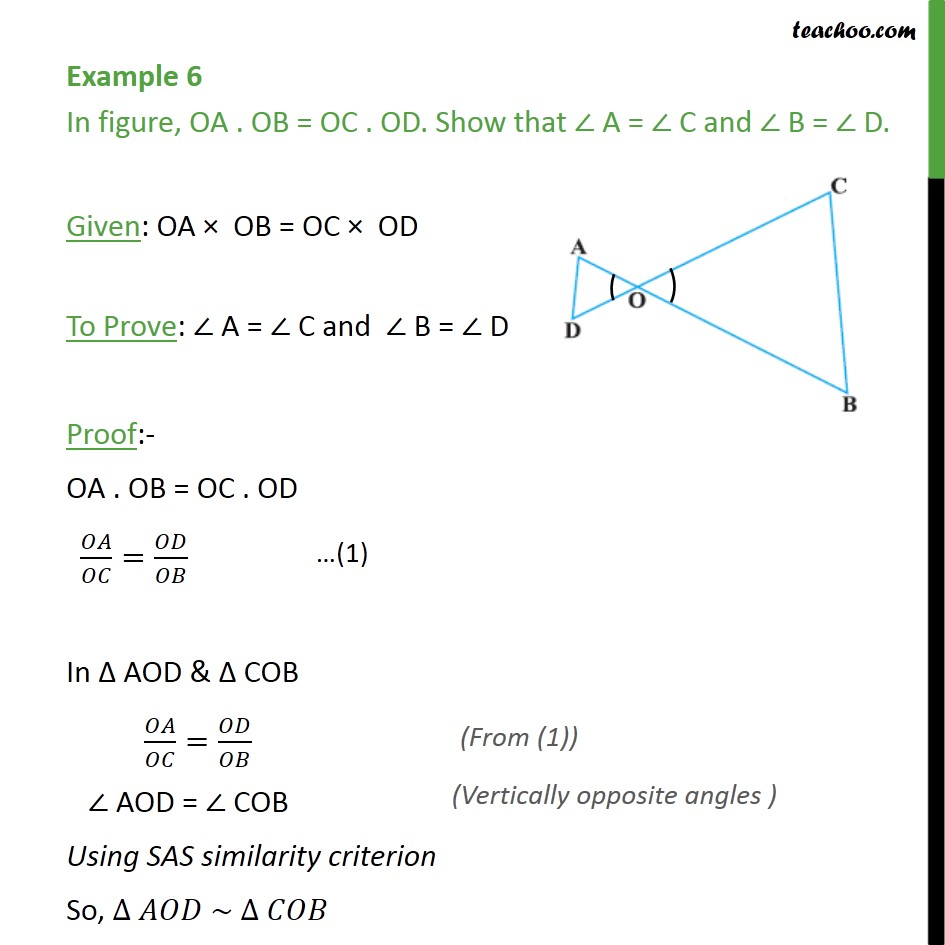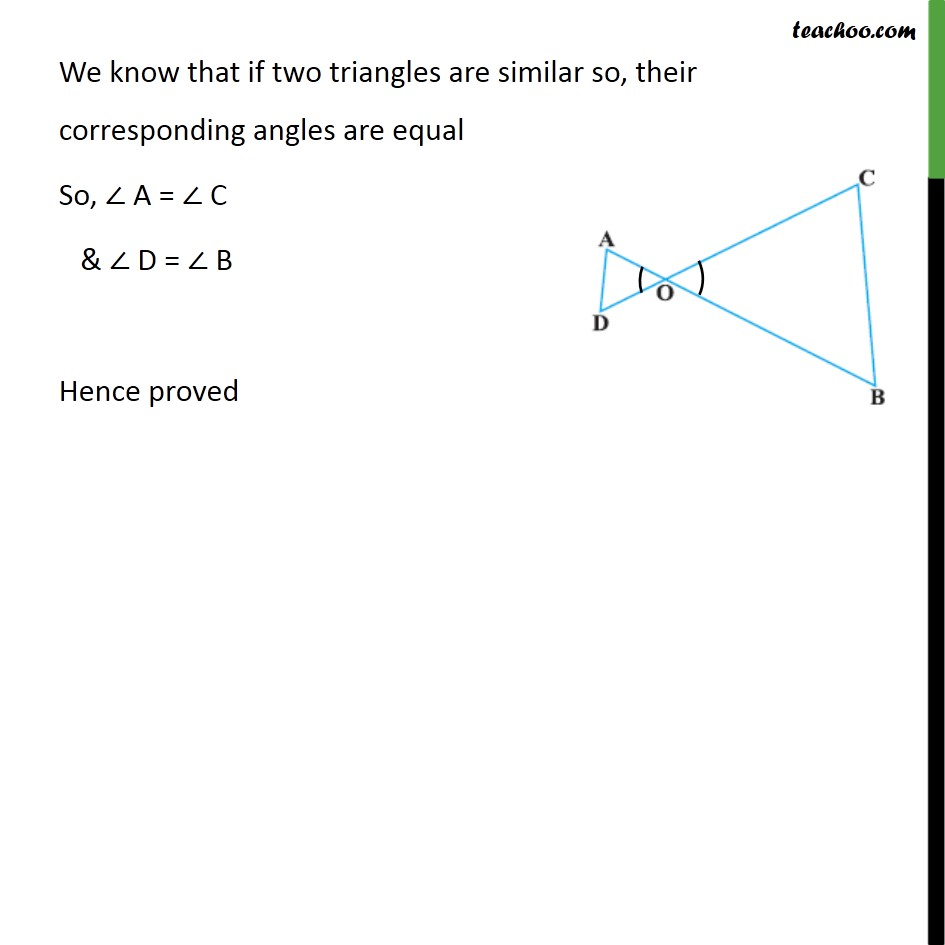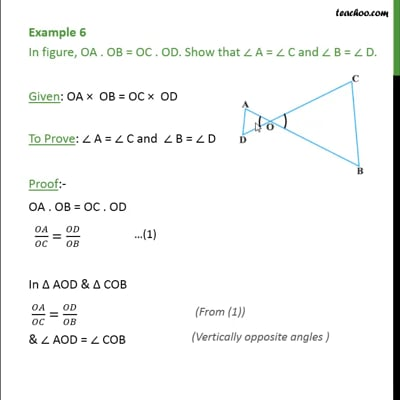Examples

Chapter 6 Class 10 Triangles (Term 1)
Serial order wiseThis video is only available for Teachoo black users

### Transcript

Example 6 In figure, OA . OB = OC . OD. Show that ∠ A = ∠ C and ∠ B = ∠ D. Given: OA × OB = OC × OD To Prove: ∠ A = ∠ C and ∠ B = ∠ D Proof:- OA . OB = OC . OD 𝑂𝐴/𝑂𝐶=𝑂𝐷/𝑂𝐵 In Δ AOD & Δ COB 𝑂𝐴/𝑂𝐶=𝑂𝐷/𝑂𝐵 ∠ AOD = ∠ COB Using SAS similarity criterion So, ∆ 𝐴𝑂𝐷 ~ ∆ 𝐶𝑂𝐵 We know that if two triangles are similar so, their corresponding angles are equal So, ∠ A = ∠ C & ∠ D = ∠ B Hence proved